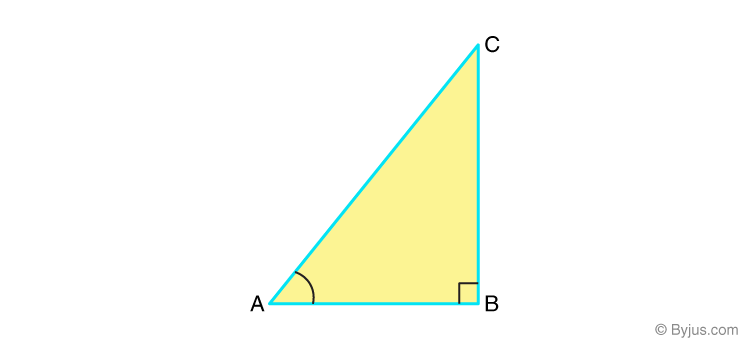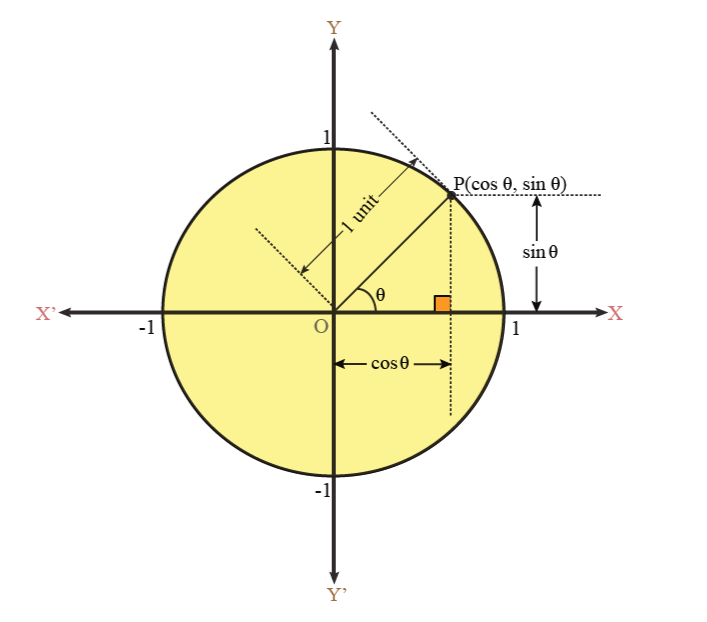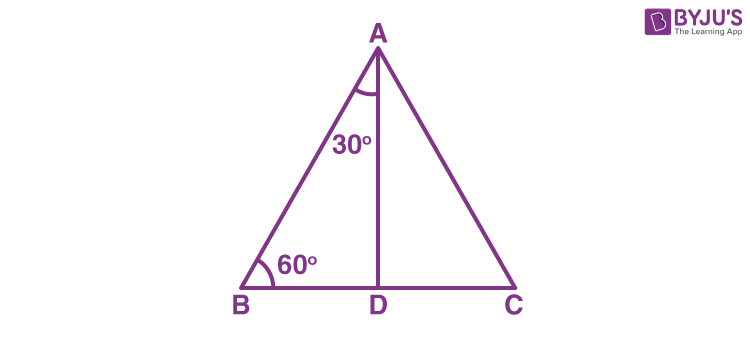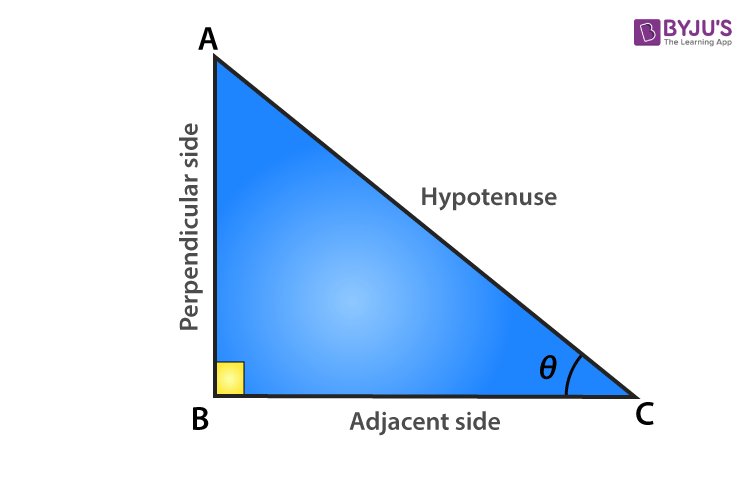Jet Set Go! All about Aeroplanes Jet Set Go! All about Aeroplanes

# Introduction To Trigonometry Class 10 Notes

## Class 10 Maths Chapter 8 Introduction to Trigonometry Notes

The notes for trigonometry class 10 Maths is provided here. In Maths, trigonometry is one of the branches, where we learn the relationships between angles and sides of a triangle. Trigonometry is derived from Greek words  ‘tri’ (means three), ‘gon’ (means sides) and ‘metron’ (means measure). In this chapter, we will learn the basics of trigonometry. Get the complete concept of trigonometry which is covered in Class 10 Maths. Also, get the various trigonometric ratios for specific angles, the relationship between trigonometric functions, trigonometry tables, various identities given here.Students can refer to the short notes and MCQ questions along with separate solution pdf of this chapter for quick revision from the links below:

## Trigonometric Ratios

### Opposite & Adjacent Sides in a Right Angled Triangle

In the ΔABC right-angled at B, BC is the side opposite to A, AC is the hypotenuse and AB is the side adjacent to A.### Trigonometric Ratios

For the right ΔABC, right-angled at B, the trigonometric ratios of the A are as follows:

• sin A=opposite side/hypotenuse=BC/AC
• cosec A=hypotenuse/opposite side=AC/BC

### Relation between Trigonometric Ratios

• cosec θ =1/sin θ
• sec θ = 1/cos θ
• tan θ = sin θ/cos θ
• cot θ = cos θ/sin θ=1/tan θ

Example: Suppose a right-angled triangle ABC, right-angled at B such that hypotenuse AC = 5cm, base BC = 3cm and perpendicular AB = 4cm. Also, ∠ACB = θ. Find the trigonometric ratios tan θ, sin θ and cos θ.

Solution: Given, in ∆ABC,

Hypotenuse, AC = 5cm

Base, BC = 3cm

Perpendicular, AB = 4cm

Then, by the trigonometric ratios, we have;

tan θ = Perpendicular/Base = 4/3

Sin θ = Perpendicular/Hypotenuse = AB/AC = ⅘

Cos θ = Base/Hypotenuse = BC/AC = ⅗

To know more about Trigonometric Ratios, visit here.

### Visualization of Trigonometric Ratios Using a Unit Circle

Draw a circle of the unit radius with the origin as the centre. Consider a line segment OP joining a point P on the circle to the centre which makes an angle θ with the x-axis. Draw a perpendicular from P to the x-axis to cut it at Q.

• sin θ=PQ/OP=PQ/1=PQ
• cos θ=OQ/OP=OQ/1=OQ
• tan θ=PQ/OQ=sin θ/cos θ
• cosec θ=OP/PQ=1/PQ
• sec θ=OP/OQ=1/OQ
• cot θ=OQ/PQ=cos θ/sin θ## Trigonometric Ratios of Specific Angles

The specific angles that are defined for trigonometric ratios are 0°, 30°, 45°, 60° and 90°.

### Trigonometric Ratios of 45°

If one of the angles of a right-angled triangle is 45°, then another angle will also be equal to 45°.Let us say, ABC is a right-angled triangle at B, such that;

∠ A = ∠ C = 45°

Thus, BC = AB = a (say)

Using Pythagoras theorem, we have;

AC2 = AB2 + BC2

= a2 + a2

= 2a2

AC = a√2

Now, from the trigonometric ratios, we have;

• sin 45° = (Opp. side to angle 45°)/Hypotenuse = BC/AC = a/a√2 = 1/√2
• cos 45° = (Adj. side to angle 45°)/Hypotenuse = AB/AC = a/a√2 = 1/√2
• tan 45° = BC/AB = a/a = 1

Similarly,

• cosec 45° = 1/sin 45° = √2
• sec 45° = 1/cos 45° = √2
• cot 45° = 1/tan 45° = 1

### Trigonometric Ratios of 30° and 60°

Here, we will consider an equilateral triangle ABC, such that;

AB = BC = AC = 2a

∠A = ∠B = ∠C = 60°

Now, draw a perpendicular AD from vertex A that meets BC at DAccording to the congruency of the triangle, we can say;

Δ ABD ≅ Δ ACD

Hence,

BD = DC

Now, in triangle ABD, ∠ BAD = 30° and ∠ ABD = 60°

Using Pythagoras theorem,

= (2a)2 – (a)2

= 3a2

So, the trigonometric ratios for 30-degree angle will be;

sin 30° = BD/AB = a/2a = 1/2

cos 30° = AD/AB = a√3/2a = √3/2

tan 30° = BD/AD = a/a√3 = 1/√3

Also,

cosec 30° = 1/sin 30 = 2

sec 30° = 1/cos 30 = 2/√3

cot 30° = 1/tan 30 = √3

Similarly, we can derive the values of trigonometric ratios for 60°.

• sin 60° = √3/2
• cos 60° = 1/2
• tan 60° = √3
• cosec 60° = 2/√3
• sec 60° = 2
• cot 60° = 1/√3

### Trigonometric Ratios of 0° and 90°

If ABC is a right-angled triangle at B, if ∠A is reduced then side AC will come near to side AB. So, if ∠ A is nearing to 0 degree, then AC becomes almost equal to AB and BC get almost equal to 0.

Hence, Sin A = BC /AC = 0

and cos A = AB/AC = 1

tan A = sin A/cos A = 0/1 = 0

Also,

cosec A = 1/sin A = 1/0 = not defined

sec A = 1/cos A = 1/1 = 1

cot A = 1/tan A = 1/0 = not defined

In the same way, we can find the values of trigonometric ratios for a 90-degree angle. Here, angle C is reduced to 0, and the side AB will be nearing side BC such that angle A is almost 90 degrees and AB is almost 0.

### Range of Trigonometric Ratios from 0 to 90 degrees

For 0∘≤θ≤90∘,

• 0 ≤ sin θ ≤ 1
• 0 ≤ cos θ ≤ 1
• 0 ≤ tan θ < ∞
1 ≤ sec θ < ∞
• 0 ≤ cot θ < ∞
• 1 ≤ cosec θ < ∞

tan θ and sec θ are not defined at  90∘.

cot θ and cosec θ are not defined at 0∘.

### Variation of trigonometric ratios from 0 to 90 degreesAs θ increases from 0 to 90

• siθ increases from 0 to 1
• coθ decreases from 1 to 0
• taθ increases from 0 to
• coseθ decreases from to 1
• seθ increases from 1 to
• coθ decreases from to 0

### Standard values of Trigonometric ratios

 ∠A 0o 30o 45o 60o 90o sin A 0 1/2 1/√2 √3/2 1 cos A 1 √3/2 1/√2 1/2 0 tan A 0 1/√3 1 √3 not defined cosec A not defined 2 √2 2/√3 1 sec A 1 2/√3 √2 2 not defined cot A not defined √3 1 1/√3 0

To know more about Trigonometric Ratios of Standard Angles, visit here.

## Trigonometric Ratios of Complementary Angles

### Complementary Trigonometric ratios

If θ is an acute angle, its complementary angle is 90θ. The following relations hold true for trigonometric ratios of complementary angles.

• si(90°− θcoθ
• co(90°− θsiθ
• ta(90°− θcoθ
• co(90°− θtaθ
• cose(90°− θseθ
• se(90°− θcoseθ

Example: Find the value of sin65°/cos25°.

Solution: Since,

cos A = sin (90° – A)

cos 25° = sin (90° – 25°)

= sin 65°

Hence, sin65°/sin65° = 1

To know more about Trigonometric Ratios of Complementary Angles, visit here.

## Trigonometric Identities

The three most important trigonometric identities are:

• sin2θ+cos2θ=1
• 1+cot2θ=coesc2θ
• 1+tan2θ=sec2θ

Example: Prove that sec A (1 – sin A)(sec A + tan A) = 1.

Solution: We will start solving for LHS, to get RHS.

sec A (1 – sin A)(sec A + tan A) = (1/cos A)(1 – sin A)(1/cos A + sin A/cos A)

= [(1 – sin A)(1 + sin A)]/cos2 A

= [1 – sin2A]/cos2A

= (cos2A)/(cos2A)

= 1

Hence proved.

To know more about Trigonometric Identities, visit here.

## Trigonometry for Class 10 Solved Problems

Example 1:

Find Sin A and Sec A, if 15 cot A = 8.

Solution:

Given that 15 cot A = 8

Therefore, cot A = 8/15.

We know that tan A = 1/ cot A

Hence, tan A = 1/(8/15) = 15/8.

Thus, Side opposite to ∠A/Side Adjacent to ∠A = 15/8

Let BC be the side opposite to ∠A and AB be the side adjacent to ∠A and AC be the hypotenuse of the right triangle ABC respectively.

Hence, BC = 15x and AB = 8x.Hence, to find the hypotenuse side, we have to use the Pythagoras theorem.

(i.e) AC2 = AB2 + BC2

AC2 = (8x)2+(15x)2

AC2 = 64x2+225x2

AC2 = 289x2

AC = 17x.

Therefore, the hypotenuse AC = 17x.

Finding Sin A:

We know Sin A = Side Opposite to ∠A / Hypotenuse

Sin A = 15x/17x

Sin A = 15/17.

Finding Sec A:

To find Sec A, find cos A first.

Thus, cos A = Side adjacent to ∠A / Hypotenuse

Cos A = 8x/17x

We know that sec A = 1/cos A.

So, Sec A = 1/(8x/17x)

Sec A = 17x/8x

Sec A = 17/8.

Therefore, Sin A = 15/17 and sec A = 17/8.

Example 2:

If tan (A+ B) =√3, tan (A-B) = 1/√3, then find A and B. [Given that  0° <A+B ≤ 90°; A>B ]

Solution:

Given that

Tan (A+B) = √3.

We know that tan 60 = √3.

Thus, tan (A+B) = tan 60° = √3.

Hence A+B= 60° …(1)

Similarly, given that,

Tan (A-B) = 1/√3.

We know that tan 30° = 1/√3.

Thus, tan (A-B) = tan 30° = 1/√3.

Hence, A-B = 30° …(2)

Now, adding the equations (1) and (2), we get

A+B+A-B = 60° + 30°

2A = 90°

A = 45°.

Now, substitute A = 45° in equation (1), we get

45° +B = 60°

B = 60°- 45°

B = 15°

Hence, A = 45 and B = 15°.

## Video Lesson on TrigonometryStay tuned with BYJU’S – The Learning App and download the app to learn all Maths-related concepts easily by exploring more videos.

Test your Knowledge on Trigonometry For Class 10

1. Shashank V M

NCERT

2. Shalini kumari

Very nice notes .It’s really help me

3. Yugansh

Can u answer my questions of this chapter!?

• Pramit Ranjan

What’s the question

4. found these points becoming most helpful to solve my confusion
thank you byju’s

5. SolangeloIsGOALSFIGHTME

Thank you!! This helped!

6. Piyush Singh

SinA=45°
CosA=35°
TanA=69°